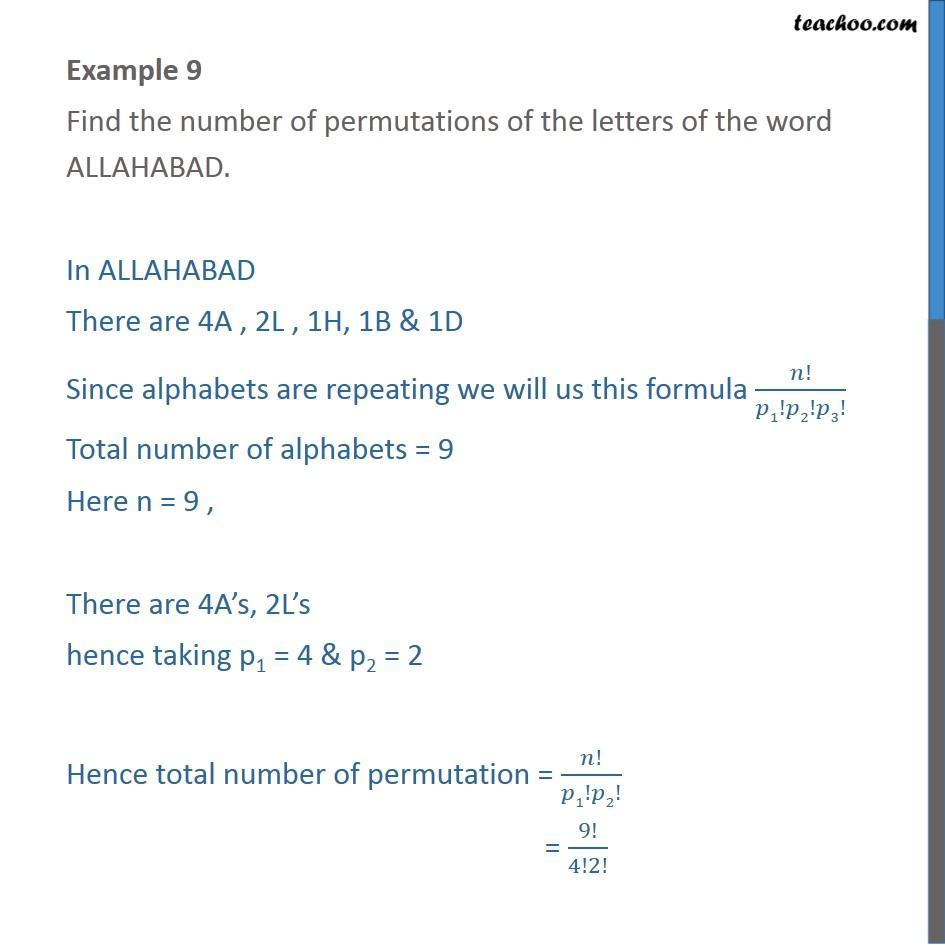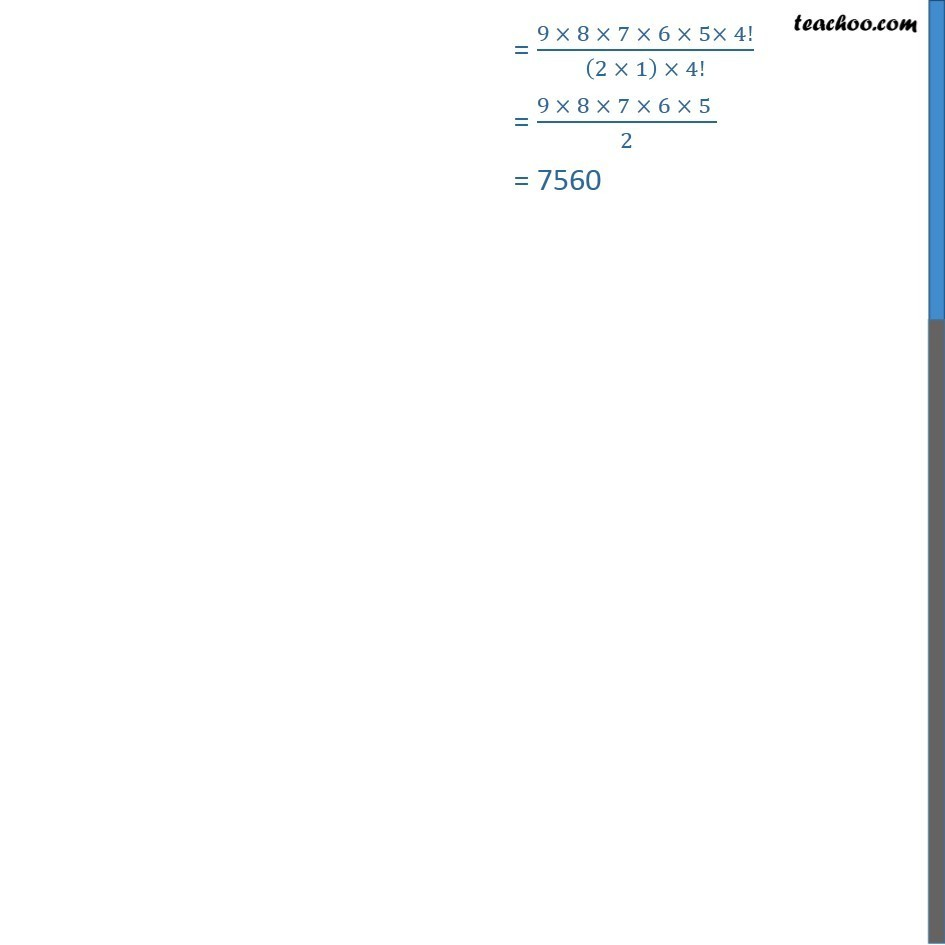Subscribe to our Youtube Channel - https://you.tube/teachoo

1. Chapter 7 Class 11 Permutations and Combinations
2. Serial order wise
3. Examples

Transcript

Example 9 Find the number of permutations of the letters of the word ALLAHABAD. In ALLAHABAD There are 4A , 2L , 1H, 1B & 1D Since alphabets are repeating we will us this formula !/ 1! 2! 3! Total number of alphabets = 9 Here n = 9 , There are 4A s, 2L s hence taking p1 = 4 & p2 = 2 Hence total number of permutation = !/ 1! 2! = 9!/4!2! = (9 8 7 6 5 4!)/((2 1) 4!) = (9 8 7 6 5 )/2 = 7560

Examples